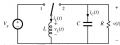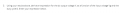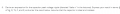# Buck-Boost homework

#### BitsNBytes

Joined Mar 22, 2021
35
Hello All,

I have a homework question that is actually related to the following threads (some were never answered, alas):

This homework is from the same course, and I need some guidance. The following questions are related, or I think related to one another.

The circuit in question:The questions in question:Per my attached work, I have derived the answer to #3 and it is correct.

I derived the answer to #6, but as a function of V, not Vg per the question. Shouldn't I be able to simply substitute the expression of V which is a function of Vg from #3 into #6? I cannot get the answer. So either my derivation of #6 is wrong, or I can't do algebra!

Guidance will greatly be appreciated.

#### Attachments

• 769.3 KB Views: 12
• 480.7 KB Views: 12
Last edited by a moderator:

#### MrAl

Joined Jun 17, 2014
10,925
Hello All,

I have a homework question that is actually related to the following threads (some were never answered, alas):

This homework is from the same course, and I need some guidance. The following questions are related, or I think related to one another.

The circuit in question:
View attachment 233377

The questions in question:
View attachment 233378
View attachment 233376

Per my attached work, I have derived the answer to #3 and it is correct.

I derived the answer to #6, but as a function of V, not Vg per the question. Shouldn't I be able to simply substitute the expression of V which is a function of Vg from #3 into #6? I cannot get the answer. So either my derivation of #6 is wrong, or I can't do algebra!

Guidance will greatly be appreciated.
If you calculate the average and you calculate the peaks based on the average then you can usually just insert the average into the expression for the peaks and that allows you to calculate the peak tp peak voltage, and then you can calculate the peak if you want.
The averaging and peak to peak expressions derived from averaging techniques are usually good enough, but they usually depend on the time constants of the circuit though to match the full blown time domain expressions.
It is instructive to derive the average and peaks using both averaging techniques and pure time domain techniques.
An averaging technique example is found here:
https://en.wikipedia.org/wiki/RC_circuit#PWM_Averaging_Responses
The example is for an unloaded RC circuit with PWM but the technique is the same for any circuit like these.

I did not go over your results yet though.

[LATER]
I got what you got for the 'inductor voltage' but i calculated it as the average capacitor voltage and so i am not entirely sure if that is what you meant by "inductor voltage".
If you had shown more of your work i would be more sure of your results, being right or wrong.

The rationale for being able to insert the average calculations into the deviation equations to get the peak to peak values is that when we were developing the average expressions from the deviation equations we were assuming all along that the variables for current and voltage always represented their average values.

Last edited:

#### BitsNBytes

Joined Mar 22, 2021
35
I should have clarified that both answers are present:
1. The inductor voltage expressed as the difference between Vg and V with D and (1-D) present in the equations.
2. The output voltage, expressed as V.

I do not understand the rest of your post, but I went back and I can finally see what I did wrong. The derivation of peak capacitor voltage ripple is correct, but I was using the equation from a Boost converter. Since this is a buck-boost, and I forgot to state that the output voltage for this circuit was given as -5V, I ultimately missed a negative sign.

#### BitsNBytes

Joined Mar 22, 2021
35
Bah! I cannot edit:

#### MrAl

Joined Jun 17, 2014
10,925
Bah! I cannot edit:

Yes that is correct for the peak to peak voltage across the capacitor. It would have been interesting to see your work too though.

It is also interesting to work up the exact time domain solutions and prove that peak to peak voltage result is correct to within some limits depending on the value of R*C and the total time period, but if the average does not depend on R and C then the time domain expressions for the average boil down to the same expressions we get from using the derivatives and the averaging techniques.
As you probably know, the buck output average voltage is D*Vg, but doing the time domain expressions is interesting because you end up with these exponentials and you have to integrate and other things, and once you simplify all that stuff it also comes out to D*E. That's the same with the single R single C PWM circuit i linked to earlier.

#### MrAl

Joined Jun 17, 2014
10,925

Doing a time domain analysis i got the following results:
iL=-2.025000416153141
vL=-10.000252080796
iH=-1.975000416153405
vH=-9.999752080692071

where iL is the lowest inductor current and iH is the highest, and
vL is the lowest cap voltage and vH the highest.
Because we know vH and vL then the peak to peak voltage is simply vH-vL. The result of this is:
Vpp=0.0005 to about 6 digits but that somewhat low number of digits is due to the subtraction of two numbers that were correct to about 13 digits.
The result of the simpler averaging technique is Vpp=0.0005 exactly.
For the inductor current, Ipp with the averaging technique was 0.05 amps and from the above doing the subtraction again we get very nearly the same result 0.05 amps to about 11 digits.
The reason for the tiny differences could be because of numerical rounding, but that could be because i chose such a small time period relative to the time constant(s) of the circuit. A larger time period with the same component values would probably result in a bigger difference, possibly much larger.
Obtaining the average however should come out to the same values for either method, except maybe for some intermediate numerical rounding effects on the results.
The values used in the circuit were:
Vin=10 Volts DC
L1=0.1 Henries
R1=10 Ohms
total time period=0.001 seconds
duty cycle=50 percent

The time domain solution is a LOT more complicated and the comparison between that and the averaging techniques really shows the beauty and simplicity of the averaging techniques.

#### MrAl

Joined Jun 17, 2014
10,925
I should have mentioned that if the solution is 2nd order the substitution of the calculated average values may not turn up the best results for the solution to some problems because the output looks too smooth. In that case you would have to use another method such as a true time domain solution of some sort. For these simpler switching circuits that usually isnt too hard to do.